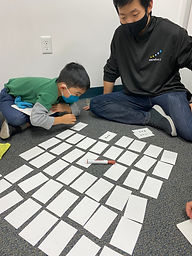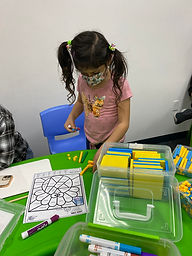## Ms. Briana

### Target 1​

###### Lesson Type:

New

Number Operation

:

Operational Relationships

Use properties of operations as strategies for solving arithmetic problems.

###### 1:

Identify the Commutative Property of Multiplication.

###### 2:

Understand that changing the order of factors, does not change the product.

###### 3:

Understand that multiplication and addition are commutative.

3rd

###### Vocabulary:

Cummutative Property, Factors, Product

Activities:

Students attempted switching the order of different operational equations to see if they maintained the same answer.

Students then determined addition and multilication are Commutative but subtraction is not.

Students played a commutative matching game to reinforce the concept.### Home Exploration

###### Guiding Questions:## Absent Students:

Avery

### Target 2

:

###### 1:

Understand that multiplying numbers makes numbers larger.

###### 2:

Understand that multiplication represents the combination of equal groups of objects.

###### 3:

Understand that multiplication can be represented with arrays, repeated addition, and skip counting.

###### 4:

Understand that the same process is utilized to multiply 3 or more whole numbers that is used to multiply only two numbers (i.e., 2x3x4 = 2 groups of 3 in 6, and 4 groups of 6 is 24).

3rd

###### Vocabulary:

Computation

Activities:

Students used dice to create 3 whole numbers for multiplication. Students then worked together to label number of groups and quantity inside of the groups to helpmsolve.

Students then used base 10 blocks to help them solve 3 whole number multiplication equations.### Home Exploration

###### Guiding Questions:### Target 3

:

###### Vocabulary:

Activities:### Home Exploration